# NCERT Math notes For Class 12 Integrals Download in PDF Chapter 7

NCERT Math Notes For Class 12 Integrals Download In PDF Chapter 7

Chapter 7: Integrals

## Chapter 7: Integrals

Let f(x) be a function. Then, the collection of all its primitives is called the indefinite integral of f(x) and is denoted by ∫f(x)dx. Integration as inverse operation of differentiation. If d/dx {φ(x)) = f(x), ∫f(x)dx = φ(x) + C, where C is called the constant of integration or arbitrary

Symbols f(x) → Integrand
f(x)dx → Element of integration
∫→ Sign of integral
φ(x) → Anti-derivative or primitive or integral of function f(x)
The process of finding functions whose derivative is given, is called anti-differentiation or integration.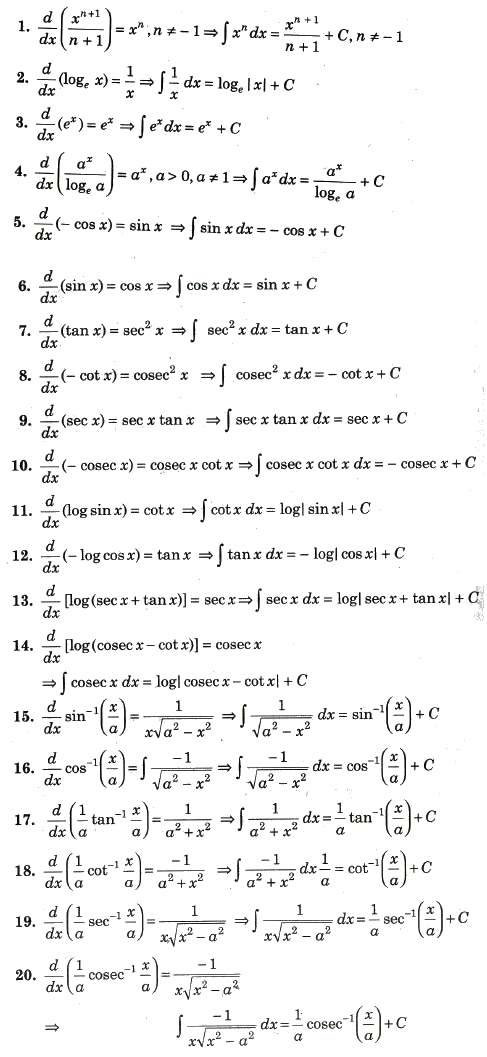### Geometrical Interpretation of Indefinite Integral

If d/dx {φ(x)} = f (x), then ∫f(x)dx = φ(x) + C. For different values of C, we get different functions, differing only by a constant. The graphs of these functions give us an infinite family of curves such that at the points on these curves with the same x-coordinate, the tangents are parallel as they have the same slope φ'(x) = f(x).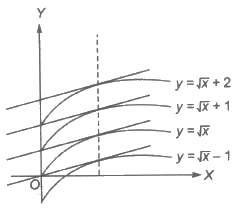Consider the integral of 1/2√x
i.e., ∫1/2√xdx = √x + C, C ∈ R

Above figure shows some members of the family of curves given by y = + C for different C ∈ R.

#### Comparison between Differentiation and Integration

(i) Both differentiation and integration are linear operator on functions as

d/dx {af(x) ± bg(x)} = a d/dx{f(x) ± d/dx{g(x)} and ∫[a.f(x) ± b.g(x)dx = a ∫f(x)dx ± b ∫g(x)dx

(ii) All functions are not differentiable, similarly there are some function which are not integrable.
(iii) Integral of a function is always discussed in an interval but derivative of a function can be
(iv) Geometrically derivative of a function represents slope of the tangent to the graph of function at the point. On the other hand, integral of a function represents an infinite family of curves placed parallel to each other having parallel tangents at points of intersection of the curves with a line parallel to Y-axis.

##### Rules of Integration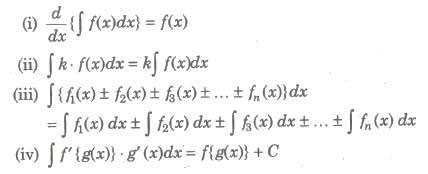##### Method of Substitution#####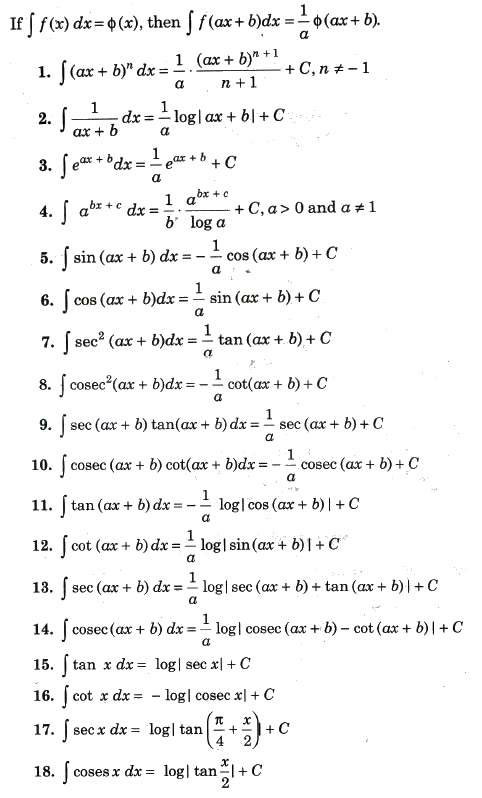If degree of the numerator of the integrand is equal to or greater than that of denominator divide the numerator by the denominator until the degree of the remainder is less than that of denominator i.e.,
(Numerator / Denominator) = Quotient + (Remainder / Denominator)

##### Trigonometric Identities Used for Conversion of Integrals into the Integrable Forms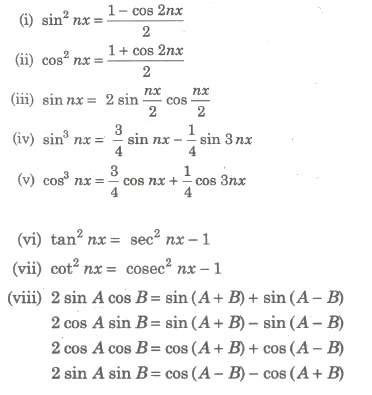##### Standard Substitution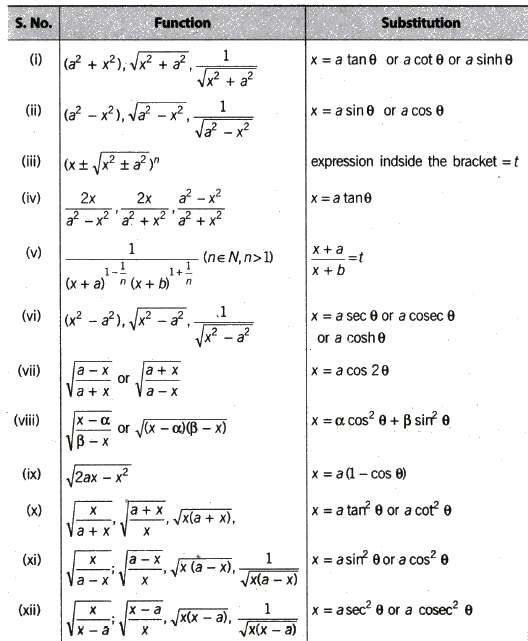#### Special Integrals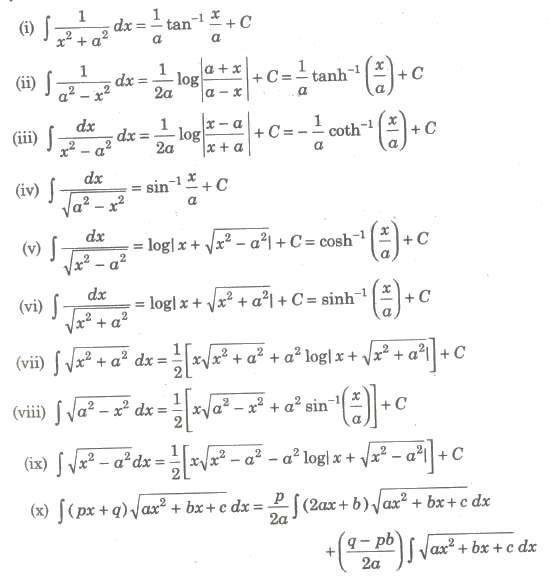##### Important Forms to be converted into Special Integrals
###### (i) Form I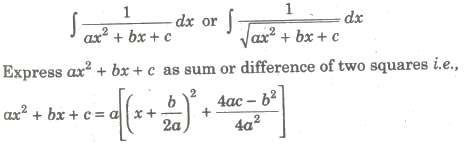###### (ii) Form II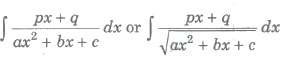Put px + q = λd / dx (ax2 + bx + c) + mu; Now, find values of λ and mu; and integrate.

###### (iii) Form III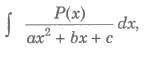when P(x) is a polynomial of degree 2 or more carry out the dimension and express in the form P(x) / (ax2 + bx + c) = Q(x) + R(x) / (ax2 + bx + c), where R(x) is a linear expression or constant, then integral reduces to the form discussed earlier.

###### (iv) Form IV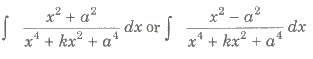After dividing both numerator and denominator by x2, put x – a2 / x = t or x + (a2 / x) = t.

###### (v) Form V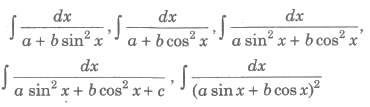To evaluate the above type of integrals, we proceed as follow (a) Divide numerator and denominator by cos2x. (b) Rreplace sec2x, if any in denominator by 1 + tan2 x. (c) Put tan x = t, then sec2xdx = dt

###### (vi) Form VI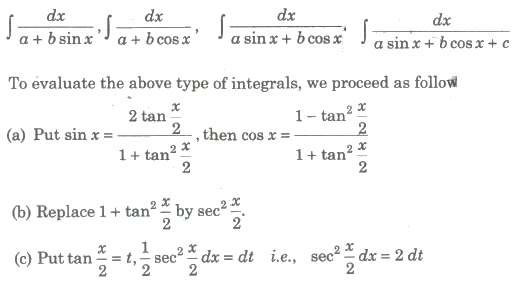###### (vii) Form VII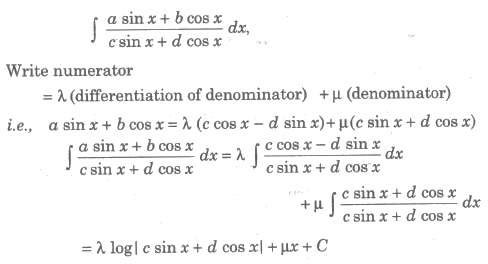###### (viii) Form VIII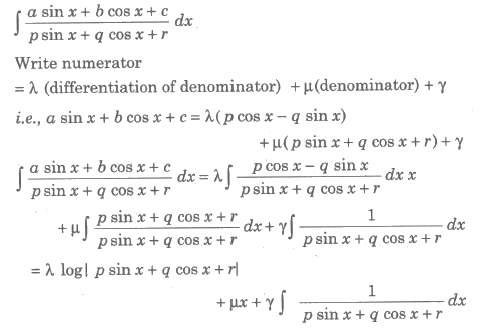###### (ix) Form IX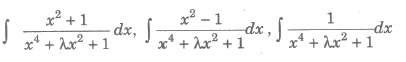To evaluate the above type of integrals, we proceed as follows

• Divide numerator and denominator by x2
• Express the denominator of integrands in the form of (x + 1/x)2 ± k2
• Introduce (x + 1/x) or d (x – 1/x) or both in numerator.
• Put x + 1/x = t or x – 1/x = t as the case may be.
• Integral reduced to the form of ∫ 1 / x2 + a2dx or ∫ 1 / x2 + a2dx

###### (x) Form X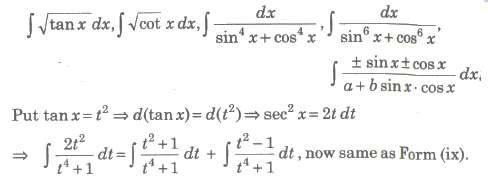##### Integration by Parts

This method is used to integrate the product of two functions. If f(x) and g(x) be two integrable functions, then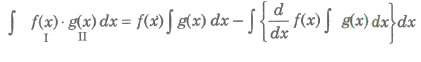(i) We use the following preferential order for taking the first function. Inverse→ Logarithm→ Algebraic → Trigonometric→ Exponential. In short we write it HATE.
(ii) If one of the function is not directly integrable, then we take it a the first function.
(iii) If only one function is there, i.e., ∫log x dx, then 1 (unity) is taken as second function.
(iv) If both the functions are directly integrable, then the first function is chosen in such a way that its derivative vanishes easily or the function obtained in integral sign is easilY integrable.

##### Integral of the Form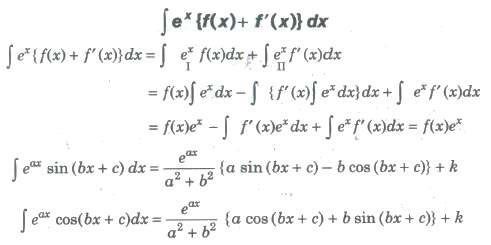##### Integration Using Partial Fractions

(i) If f(x) and g(x) are two polynomials, then f(x) / g(x) defines a rational algebraic function of x. If degree of f(x) < degree of g(x), then f(x) / g(x) is called a proper rational function.
(ii) If degree of f(x) ≥ degree of g(x), then f(x) /g(x) is called an improper g(x) rational function.
(iii) If f(x) / g(x) isan improper rational function, then we divide f(x) by g(x) g(x) and convert it into a proper rational function as f(x) / g(x) = φ(x) + h(x) / g(x).
(iv) Any proper rational function f(x) / g(x) can be expressed as the sum of rational functions each having a simple factor of g(x). Each such fraction is called a partial fraction and the process of obtaining them, is called the resolution or decomposition of f(x) /g(x) partial fraction.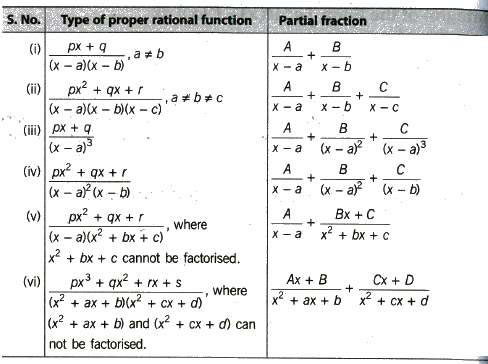##### Shortcut for Finding Values of A, B and C etc.

Case I. When g(x) is expressible as the product of non-repeated line factors.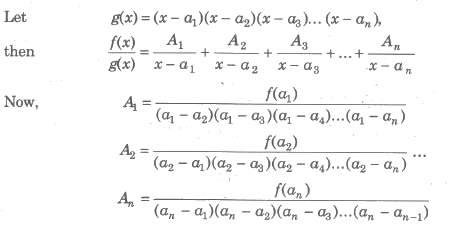Trick To find Ap put x = a in numerator and denominator after P deleting the factor (x — ap).

Case II. When g(x) is expressible as product of repeated linear factors.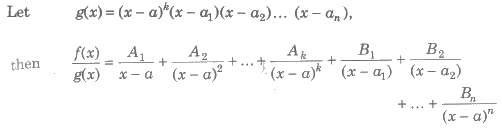Here, all the constant cannot be calculated by using the method in Case I. However, Bl, B2, B3, … , Bn can be found using the same method i.e., shortcut can be applied only in the case of non-repeated linear factor.

##### Integration of Irrational Algebraic Function

Irrational function of the form of (ax + b)1/n and x can be evaluated by substitution (ax + b) = tn, thus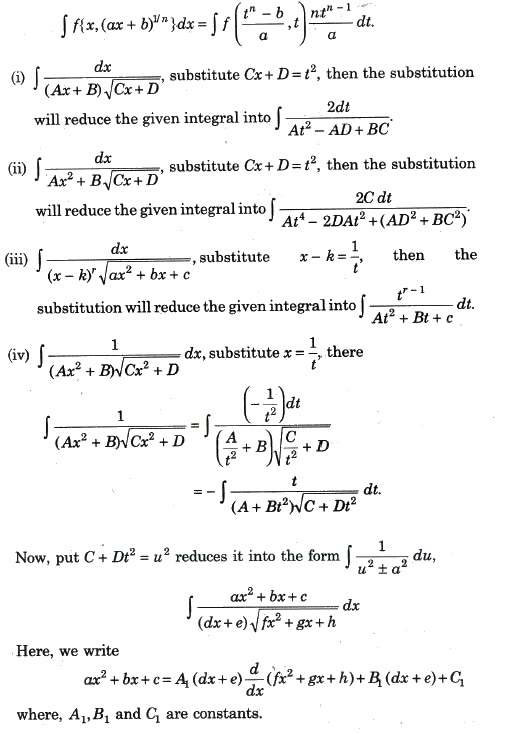Integrals of the Type (bxm + bxn)P

Case I. If P ∈ N (natural number) we expand the binomial theorem and integrate.

Case II. If P ∈ Z (integers), put x = pk, where k denominator of m and n.

Case III. If (m+1)/n is an integer, we put (a + bxn) = rk, where k is th denominator of the fraction.

##### Integration of Hyperbolic Functions

• ∫sinh x dx = cosh x + C
• ∫cosh x dx = sinh x + C
• ∫sech2x dx = tanh x + C
• ∫cosech2x dx = – coth x + C
• ∫sech x tanh x dx = – sech x + C
• ∫cosech x coth x dx = – cosech x + C

Case IV If {(m+1)/n} + P is an integer, we put (a + bxn) = rkxn is the denominator of the fraction p.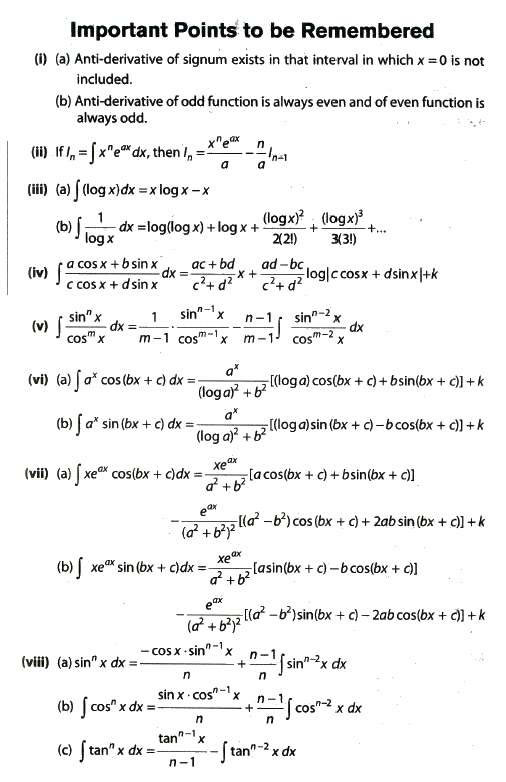### NCERT Books Free Pdf Download for Class 5, 6, 7, 8, 9, 10 , 11, 12 Hindi and English Medium

 Mathematics Biology Psychology Chemistry English Economics Sociology Hindi Business Studies Geography Science Political Science Statistics Physics Accountancy